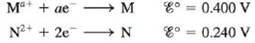Chapter 18, Problem 156IP

Chapter
Section
Textbook Problem

# An electrochemical cell is set up using the following unbalanced reaction: M a + ( a q ) + N ( s ) → N 2 + ( a q ) + M ( s ) The standard reduction potentials are:The cell contains 0.10 M N2+ and produces a voltage of 0.180 V. If the concentration of Ma+ is such that the value of the reaction quotient Q is 9.32 × 10−3, calculate [Ma+]. Calculate wmax for this electrochemical cell.

Interpretation Introduction

Interpretation: The concentration of [Ma+] and wmax of the given electrochemical cell is to be calculated.

Concept introduction: Electrochemical cell is defined as a cell that generates electrical energy from a redox reaction taking place in a cell. Nernst equation used in an electrochemical cell is used to relate the standard reduction potential, reduction potential, activities and temperature in a redox reaction.

To determine: The concentration of [Ma+] and wmax of the given electrochemical cell.

Explanation

Explanation

Given

The concentration of N2+ is 0.10 M .

Ecell=0.180 V

Reaction quotient Q 0r[a1]  equilibrium constant is 9.32×103 .

The given reaction is,

Ma++NN2++M

The reaction quotient of the reaction is given as,

[N2+][Ma+]=Q .

The concentration of [Ma+] is calculated as per data,

[N2+][Ma+]=9.32×103 .

The concentration of N2+ is 0.10 M .

Substitute the value of N2+ in the above expression.

[N2+][Ma+]=9.32×103[0.1 M][Ma+]=9.32×103[Ma+]=0

### Still sussing out bartleby?

Check out a sample textbook solution.

See a sample solution

#### The Solution to Your Study Problems

Bartleby provides explanations to thousands of textbook problems written by our experts, many with advanced degrees!

Get Started